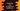# Learn to implement bubble sort in JavaScript## How to implement bubble sort in JavaScript:

Bubble sort is a sorting algorithm that sorts all elements of a list by comparing and swapping all pairs of adjacent elements. This is not the best sorting algorithm and its complexity is Ο(n2) for worst case scenario.

In this post, we will learn how to implemtn bubble sort in JavaScript with example.

### How Bubble sort works:

Let’s consider the below list :

``[10,2,13,40,9]``

#### Loop 1:

In this loop, we will check the first and the second element and swap them if the second element is smaller than the first. It will become:

``[2,10,13,40,9]``

Next, check for second and third and swap them if required:

``[2,10,13,40,9]``

No swapping is required this time.

Similarly, check for third and fourth :

``[2,10,13,40,9]``

No swapping is required. and finally check for the last two :

``[2,10,13,9,40]``

Swapping is required. So, after the first loop is done, the largest element is moved to the end of the list. Similarly, after the second iteration, the second largest element is moved to end, on third iteration the third largest element is moved to end etc.

### Bubble sort in JavaScript:

Let’s write the bubble sort in JavaScript:

``````const swap = (arr, i, j) => {
let temp = arr[i];
arr[i] = arr[j];
arr[j] = temp;
};

const bubbleSort = (arr) => {
let len = arr.length;
let i, j, end;

for (i = 0; i < len; i++) {
end = len - i;
for (j = 0; j < end; j++) {
if (arr[j] > arr[j + 1]) {
swap(arr, j, j + 1);
}
}
}
return arr;
};

let givenArray = [2, 30, 13, 20, 11, 14, 12, 100];
console.log(bubbleSort(givenArray));``````

### Explanation :

We have two functions in this program : swap and bubbleSort. swap function is used to swap two numbers and bubbleSort is used for sorting an array using bubble sort.

The swap method uses one temporary variable to swap two numbers. We are passing one array, and two indices to swap the numbers in this indices.

We are using two for loops to do the bubble sort.

### Output :

If you execute the above program, it will print the below output:

``````[
2, 11, 12,  13,
14, 20, 30, 100
]``````

It is the sorted array that we passed to bubbleSort.